# Intermediate Geometry : How to find the volume of a cube

## Example Questions

1 2 4 Next →

### Example Question #67 : Cubes

A sphere with a radius ofis cut out of a cube that has a side length of. What is the volume of the resulting figure?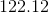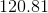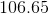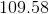Explanation:

Since the cube is bigger, we will be subtracting the volume of the sphere from the volume of the cube.

Start by recalling how to find the volume of a sphere.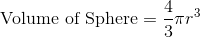Plug in the given radius to find the volume.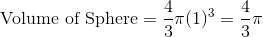Next, recall how to find the volume of a cube: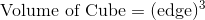Plug in the given side length to find the volume of the cube.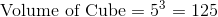Finally, subtract the volume of the sphere from the volume of the cube.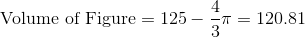Make sure to round toplaces after the decimal.

### Example Question #68 : Cubes

A sphere with a radius ofis cut out of a cube with a side length of. What is the volume of the resulting figure?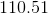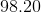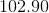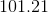Explanation:

Since the cube is bigger, we will be subtracting the volume of the sphere from the volume of the cube.

Start by recalling how to find the volume of a sphere.Plug in the given radius to find the volume.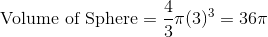Next, recall how to find the volume of a cube:Plug in the given side length to find the volume of the cube.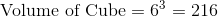Finally, subtract the volume of the sphere from the volume of the cube.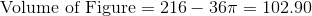Make sure to round toplaces after the decimal.

### Example Question #61 : Solid Geometry

A sphere with a radius ofis cut out of a cube with a side length of. What is the volume of the resulting figure?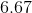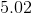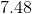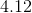Explanation:

Since the cube is bigger, we will be subtracting the volume of the sphere from the volume of the cube.

Start by recalling how to find the volume of a sphere.Plug in the given radius to find the volume.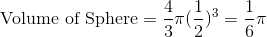Next, recall how to find the volume of a cube:Plug in the given side length to find the volume of the cube.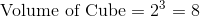Finally, subtract the volume of the sphere from the volume of the cube.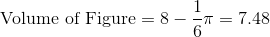Make sure to round toplaces after the decimal.

### Example Question #70 : Cubes

A sphere with a radius ofis cut out of a cube that has a side length of. What is the volume of the resulting figure?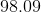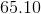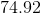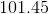Explanation:

Since the cube is bigger, we will be subtracting the volume of the sphere from the volume of the cube.

Start by recalling how to find the volume of a sphere.Plug in the given radius to find the volume.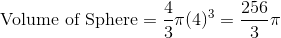Next, recall how to find the volume of a cube:Plug in the given side length to find the volume of the cube.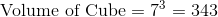Finally, subtract the volume of the sphere from the volume of the cube.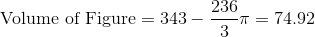Make sure to round toplaces after the decimal.

### Example Question #71 : Solid Geometry

A sphere with a radius ofis cut out of a cube that has a side length of. What is the volume of the resulting figure?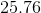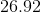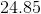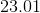Explanation:

Since the cube is bigger, we will be subtracting the volume of the sphere from the volume of the cube.

Start by recalling how to find the volume of a sphere.Plug in the given radius to find the volume.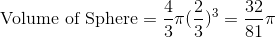Next, recall how to find the volume of a cube:Plug in the given side length to find the volume of the cube.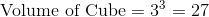Finally, subtract the volume of the sphere from the volume of the cube.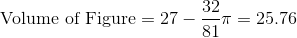Make sure to round toplaces after the decimal.

### Example Question #72 : Cubes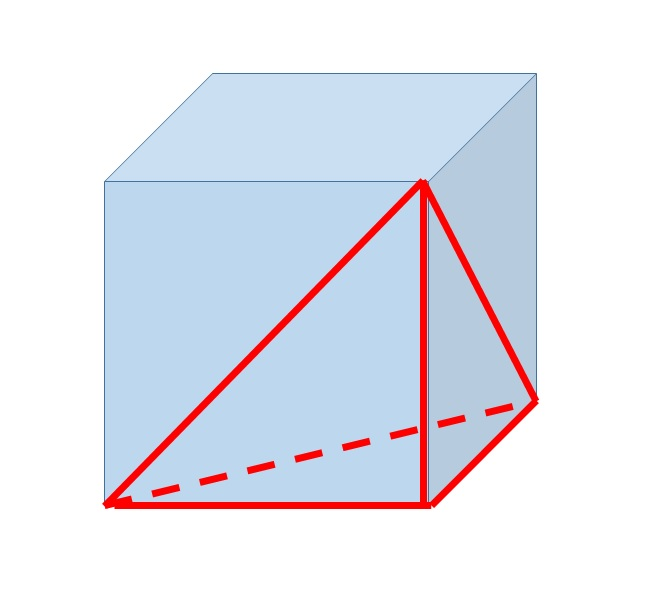The above figure shows a triangular pyramid inscribed inside a cube. The pyramid has volume 100. Give the volume of the cube.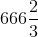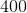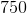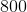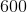Explanation:

Letbe the length of one side of the cube. Then the base of the pyramid is a right triangle with legs of length. The area of a right triangle is half the product of the lengths of the legs, so the base has area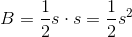The volume of a pyramid is one third the product of its height and the area of its base. The height of the pyramid is, so the volume is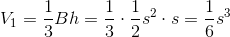The volume of the cube is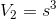, so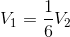,

or, equivalently,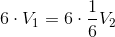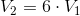That is, the volume of the cube is six times that of the pyramid, and, since the pyramid has volume 100, the volume of the cube is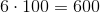.

1 2 4 Next →